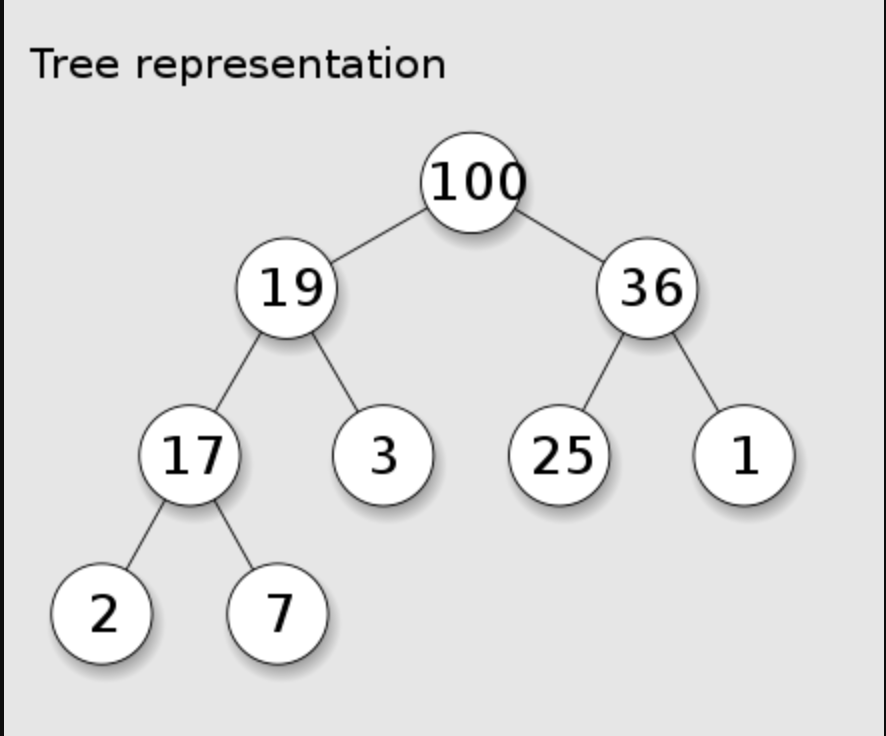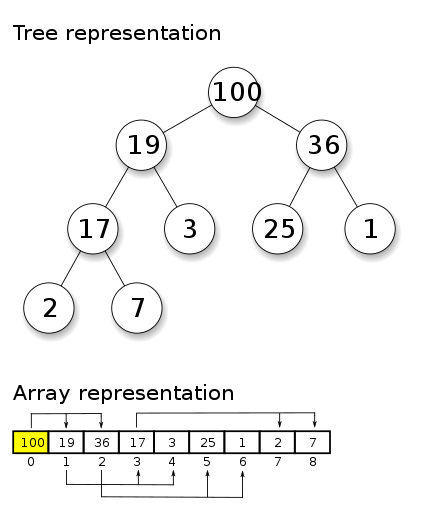<Daniel Sasse/># Algorithm Tutorial: Intro to Heaps and Priority Queue Implementation

algorithms

javascript

codenewbie

Published: Thu Jun 17 2021 (~ 8 min)

In this edition of the Algorithm Tutorial series, we're going to break down the Heap data structure and and its utilization to implement a priority queue.

## Contents

### Background

Imagine you had a list of values that you had to operate on, and needed to use the values from greatest to least or vice versa. A simple approach, would be to sort the list, and then proceed in the desired order. However, this can become more complicated if new values are continually added to the list, requiring the list to be reordered before you can proceed. Since re-sorting the listed could potentially require comparing the new value to every other entry element in the list, this can become a slow process as the list grows.

Secondly, imagine the waiting area of an emergency room. As new patients come in, they could simply be added to a queue to wait and see a doctor, however this wouldn't account for the patient's severity of symptoms. A patient suffering from a heart attack, should clearly be a higher priority than someone with a broken toe and should be helped first, even if they joined the queue last. How to we adjust our list/queue to account for priority, despite when it was added?

### Heap Structure

What makes a heap faster and more efficient than simply resorting a list over and over is its tree based structure according to its heap property (max or min). In a max heap, the root of the tree will always be the element with the maximum value being used to compare, and for each node of the tree the children of a node must be less than or equal to the value of the node.Above, we see a model of a common heap implementation called a binary heap, specifically a max heap. If we imagine a new value of 200 being added to the end of the queue (bottom of the tree), instead of comparing it to every other value as you would when sorting an array, you would only need to compare it to its parent to determine if it should be higher in the queue or remain where it is. Utilizing this, it becomes significantly more efficient to insert new values into our heap at the correct position. In terms of Big O notation, this insertion process would be modeled as O(log n) since we have to make at most one comparison per tier of the tree, whereas comparing potentially every item, O(n), if we were inserting into an already sorted list.

In terms of working with a heap, the process will vary depending on the language. Python, for example, has the heapq library which can be imported and worked with immediately, however in Javascript there is no native Heap data structure and it must be implemented manually. Let's walk through how this could be done in Javascript.

### Implementation

#### Initialization

To implement a binary max heap in Javascript, we'll start by defining a new class `MaxHeap` with a value property of an empty array. We can optionally initialize a `size` property to keep count of the number of values in our heap to improve the readability of future code instead of having to write `this.values.length` each time.

``````class MaxHeap {
constructor(){
this.values = []
this.size = 0
}
}``````

If a heap is a tree structure, why are we initializing the heap with an empty array?

Any binary tree structure can be stored as an array (as opposed to creating a Tree class) due to the relationship between the index of any single node and both of its child nodes as shown below.For any node `n`, we can calculate the index of:

• Its left child = `2 * n + 1`
• Its right child = `2 * n + 2`
• Its parent = `Math.floor( (n - 1) / 2 )`

For example, the root node has an index of 0, with its left child being `1` and its right child being `2`. Node `2`s children would be at indices `5` and `6`.

#### Inserting Values

To add values to the heap, we will add them to the next empty position in the heap. In the tree structure, this means the value will be in the bottom tier of the tree, in the left-most empty child spot. Comparing this to the array structure, we will be adding it to the end of the array( think `.push()` ). Once the value is in the heap, we need to compare it to its parent node(s) and we will swap this new node with its parent if the heap property is currently being violated.

For instance, in the previous example of inserting 200 into the max heap we would need continue swapping 200 with each parent value until it reached the root since 200 would be the largest value in the entire heap. In the case of a priority queue we would use a similar swap pattern, but we would compare whatever property we define for the priority. This process of swapping the node upwards through the heap goes by a number of names, but I will refer to it as "bubbling up".

Here is an implementation of how we can insert a new value into the heap. If more than one value is in the heap, we will `bubbleUp()`, moving the newest value to its correct position:

``````class MaxHeap {
constructor(){
this.values = []
this.size = 0
}

insert(value){
// If no value, do nothing
if (value === undefined) return
// Insert the value, and increment the size of the heap
this.values.push(value)
this.size++
// Check to see if there is not more than 1 item in the heap
// If there is only 1 item, there is no need to bubble up
if (this.size > 1) this._bubbleUp()
return this.values
}

_bubbleUp(){
// Grab the most recently added value and its parent
let currentIndex = this.size - 1
let parentIndex = Math.floor( (currentIndex - 1) / 2 )

// Swap the new node with its parent until the new node either
// becomes the root, or is no longer greater than its parent
while (parentIndex >= 0 && this.values[currentIndex] > this.values[parentIndex]){
this._swap(currentIndex, parentIndex)
currentIndex = parentIndex
parentIndex = Math.floor((currentIndex - 1) / 2 )
}
}

// Helper function using object destructuring to swap the elements at two indices
_swap(index1, index2){
[this.values[index1], this.values[index2]] = [this.values[index2], this.values[index1]]
}
}``````

Example:

``````const heap = new MaxHeap()
const values = [17,2,36,100,7,1,19,25,3,]

for (let val of values){
heap.insert(val)
}
// Resulting Heap: [100, 36, 19, 25, 7, 1, 17, 2, 3]``````

#### Extracting Values

The purpose of using a heap in this fashion, is to quickly access the max/min value (or the value with the max/mix priority) depending on whether you are using a max or min heap. Because of how it is structure and the "bubbling" mechanism, this value will always be the first item in the heap array we have created, and this is the value we want to extract.

The problem we have, is that if we simply removed the first item in an array with `unshift()`, the entire array would need to be reindexed, as each index would need to be reassigned a new value. The only way to avoid this re-indexing, is if we removed the last item in a list, which is what we will do here by swapping the first and last items in the heap and then extracting.

Initially after the swap, the rule governing the heap (max/min) will be violated, and we must restore it similar to how we "bubbled up" before. In this case, we will need to compare this new out-of-place value with each of its children, and cause it to "trickle down" until it the heap rule is restored. This process is also sometimes referred to as "sifting down". As we compare the node with each of its children, we will swap with whichever child is greater (in max heap) or lesser (in min heap).

``````class MaxHeap {
/**
*
*/

extract(){
if (this.size === 0) return
// Swap the value to be extracted (root) with the last item in the heap
const lastIndex = this.size - 1
this._swap(0, lastIndex)
// Remove the value to be extracted
const extractValue = this.values.pop()
this.size--
// If there is more than one remaining value, we must restore the heap rule
if (this.size > 1) this._trickleDown()
return extractValue
}

_trickleDown(){
let currentIndex = 0
/**
* These will be the indexes corresponding to the left and right
* child of the node at currentIndex
* swapIdx will be which of the children the currentIndex will
* actually switch with, if any
*/
let leftIdx, rightIdx, swapIdx
while (true) {
leftIdx = 2 * currentIndex + 1
rightIdx = 2 * currentIndex + 2
swapIdx = null
/**
* If there is a valid left child and it is greater than the current value,
* prepare to swap it
*/
if (
leftIdx < this.size &&
this.values[currentIndex] < this.values[leftIdx]
) {
swapIdx = leftIdx
}
/**
* If there is a valid right child and it is greater than the current value,
* prepare to swap it if we haven't already prepared to swap with left child.
* If we have prepared to swap with left child, we should only choose to swapIdx
* with the right child instead if it is greater than the left child, meaning
* it better fits the heap rule
*/
if (
rightIdx < this.size &&
((swapIdx === null &&
this.values[currentIndex] < this.values[rightIdx]) ||
(swapIdx !== null &&
this.values[rightIdx] > this.values[leftIdx]))
) {
swapIdx = rightIdx
}
if (swapIdx === null) break // If no possible swap was ID'd, we're done
// Swap the parent with the identified child, update the currentIndex, and repeat
this._swap(currentIndex, swapIdx)
currentIndex = swapIdx
}
}
}``````

Example Extraction using previously created heap:

``````heap.extract() // 100
heap.values // [36, 25, 19, 3, 7, 1, 17, 2]
heap.extract() // 36
heap.values // [25, 7, 19, 3, 2, 1, 17]
heap.extract() // 25
heap.values // [19, 7, 17, 3, 2, 1]``````

#### As a Priority Queue

In the emergency room example discussed in the introduction, it would be impractical to keep track of the order to see patients just by the order that they arrived. It makes sense then, to use a priority queue, where the next patient to be seen is the one with the most urgent needs, regardless of when they entered the queue. This is a perfect use case for a heap, but instead of each element in the heap being just a number, there will likely be other information such as a patient name or id#. In this case, when we insert the value into the heap, we could insert it as an object with a key:value pairs for the patient and the priority level. We would then need to adjust the `bubbleUp()` and `trickleDown()` methods to compare the value of the priority key for each element.

### Full Code

Combining the code above, below you will find two full samples of heap implementation. The first is for a maxHeap based on the value of the element. The second would be a possible implementation for a _maxHeap priority queue where the values will be placed according with the highest priority numbers being the first to extract.